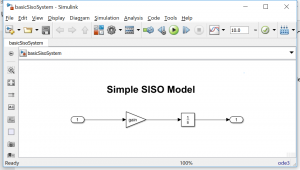# A Classy MATLAB Wrapper for your C++

Greg’s pick this week is Example MATLAB class wrapper for a C++ class by Oliver Woodford.

You have a C++ class that you would like to instantiate in MATLAB.

If you are using MATLAB R2019a or later: Check out Directly calling C++ libraries from MATLAB

If you are using R2018b or earlier release, you cannot call C++ code directly in MATLAB and it needs to be imported via a MEX-File.

However MEX-files can only provide a function interface to MATLAB, and you want to instantiate an object from a class. Now what do you do?

Oliver Woodford comes to the rescue with a splendid example of how to wrap up your C++ class so you can instantiate it and
maintain it from MATLAB.

Spoiler: I used this to export a Simulink model as a MATLAB Class.

### A Nifty MEX-wrapper to Interface With the Class

Since MEX-files only support function based interfaces, we need to create a wrapper function that enables

• Instantiating the C++ class as an object
• Calling methods on that object (or changing property values)
• Destroying the object

To employ the different methods of the C++ class, the first input argument to the MEX-function is used to identify which method
of the C++ class is to be called.

MEX-code

   void mexFunction(int nlhs, mxArray *plhs[], int nrhs, const mxArray *prhs[])
{
// Get the command string
char methodId;
mxGetString(prhs, methodId, sizeof(methodId))
mexPrintf(“***Method Id: %s\n”, methodId);
}


MATLAB Code:

>> out = myMexFunction(‘myMethod’, in);

***Method Id: myMethod

>>

The additional arguments of the MEX-function are used as inputs to the C++ class methods.

Once the MEX-function wrapper is in place and compiled, a MATLAB Class is created that mirrors the C++ class, and which uses
the MEX-function wrapper to map the various properties and methods of the MATLAB Class to the C++ class.

### Why All the Complexity? What Does this Solve?

There are three key elements that make this entry excellent.

1. It demonstrates how to use the C++ code “safely” in the context of the MEX-function
2. It includes a helper file with several utilities that make part 1 easy.
3. It provides an example to use as a template for your own project

The tricky part about using a C++ class comes when you want to have multiple instances of the class persist between calls
to the MEX-function wrapper. If we’re not careful, we could introduce memory leaks.

This is where Oliver’s entry shines. He exports a reference to the class instance back to MATLAB so its lifecycle can be
synchronized with the lifecycle of a MATLAB Class instance.

That’s what this line:

    plhs = convertPtr2Mat<CPP_CLASSNAME>(new CPP_CLASSNAME);

does when creating a new instance of the C++ class. A handle to the new object is output from the MEX-function as a 64-bit
integer.

This handle can then be used to refer to that particular instance of the C++ class from MATLAB. Therefore you see references
to

    objectHandle =  prhs;    CPP_CLASSNAME *cppObj = convertMat2Ptr<CPP_CLASSNAME>(objectHandle);

which convert the 64-bit integer handle that MATLAB uses back to the native C++ class inside the MEX-function.

### Deploy a Simulink Model as a MATLAB Class

To try out this entry, I used Simulink Coder to generate a C++ class for a Simulink modelThis simple model was configured to generate C++ as code as a C++ class.This encapsulates the generated code providing methods for initializing, stepping the model at each sample time, and terminating
the execution of the model.

I did not use the default main function, but instead copied MATLAB_ROOT/rtw/c/src/common/rt_cppclass_main.cpp as a new CPP-file and changed the main function to a mexFunction based on Oliver’s example class_interface_mex.cpp.

The corresponding MATLAB Class I wrote looked like this:

type('basicSisoSystemInterface.m')
%CLASS_INTERFACE Example MATLAB class wrapper to an underlying C++ class
classdef basicSisoSystemInterface < handle
properties (SetAccess = private, Hidden = true)
ObjectHandle; % Handle to the underlying C++ class instance
end
methods
%% Constructor - Create a new C++ class instance
function this = basicSisoSystemInterface(varargin)
this.ObjectHandle = basicSisoSystem_mex('new', varargin{:});
end

%% Destructor - Destroy the C++ class instance
function delete(this)
basicSisoSystem_mex('delete', this.ObjectHandle);
end

%% sim - an example class method call
function varargout = sim(this, varargin)
[varargout{1:nargout}] = basicSisoSystem_mex('sim', this.ObjectHandle, varargin{:});
end

end
end


Where I included a method called “sim” to execute the C++ version of the model.

Now I can call two different instances of the same model. In this case with different inputs.

modelObj1 = basicSisoSystemInterface;
modelObj2 = basicSisoSystemInterface;
out1 = sim(modelObj1, 3*ones(100, 1));
out2 = sim(modelObj2, 5*ones(100, 1));
plot(out1)
hold on
plot(out2)
title('Multiple Instance model outputs')
xlabel('Time (ms)')
ylabel('Model Output')
legend('Model Instance 1', 'Model Instance 2')
grid on

### What do you think?

Let us know here.

Published with MATLAB® R2017b

|

### 댓글

댓글을 남기려면 링크 를 클릭하여 MathWorks 계정에 로그인하거나 계정을 새로 만드십시오.